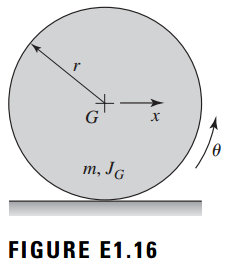### Create an Account

Home / Questions / Consider the disc rolling along a line in Figure E116 The disc has a mass m and a rotary i...

# Consider the disc rolling along a line in Figure E116 The disc has a mass m and a rotary inertia JG about the center of mass G Answer the following How many degrees of freedom does this system

Consider the disc rolling along a line in Figure E1.16. The disc has a mass m and a rotary inertia JG about the center of mass G. Answer the following: (a) How many degrees of freedom does this system have? and (b) Determine the kinetic energy for this systemMay 16 2020 View more View LessSubscribe To Get Solution